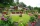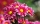# A rectangular 4

A rectangular garden has a length of 57 m and a width of 42 m. Calculate of how many m2 will decrease the area of a garden, if the ornamental fence with a width of 60 cm will be planted inside its perimeter.

S =  117.36 m2

### Step-by-step explanation:Did you find an error or inaccuracy? Feel free to write us. Thank you!#### You need to know the following knowledge to solve this word math problem:

We encourage you to watch this tutorial video on this math problem:

## Related math problems and questions:

• GardenThe rectangular garden has a length 99 m and width 84 m. Calculate how many m2 will decrease its area if land by the ornamental fence with width 30 cm.
• Decorative fenceThe rectangular garden is 57 m long and 42 m wide. Calculate how much m² it will reduce if it encloses with a 60 cm wide decorative fence.
• Rectangle 45The perimeter of a rectangle is 60cm. If the length of the rectangle is 20cm. a)find the width b)find the area.
• Rectangular gardenRectangular garden has a length of 48.7 m, a width of 6.3 meters shorter than the length. How much mesh should be bought for its fencing if the gate is 2.9 m long and the gate 1.1 m? What is the area of the garden?
• TableclothsThe restaurant has sixty-two square tablecloths with a side length of 150 cm and 36 rectangular tablecloths with dimensions of 140 cm and 160 cm. A) How many meters of hemming ribbon will be needed if we add 50 cm to each tablecloth? B) The ribbon sale in
• Rectangular garden 2A farmer bought 600 m of wire for the fence. He wants to use it to besiege a rectangular garden with a surface of 16875 m2. Calculate the size of the garden.
• Rectangular gardenThe perimeter of Peter's rectangular garden is 98 meters. The width of the garden is 60% shorter than its length. Find the dimensions of the rectangular garden in meters. Find the garden area in square meters.
• Garden plotCalculate how many meters of fence need to fence the square garden with length and width of 12 meters.
• Area and perimeter 2Find the area and the perimeter of a rectangle of length 45 1/2 cm and breadth 16 2/3 cm.
• GardensThe area of the square garden is 3/4 of the area of the triangular garden with sides of 80 m, 50 m, 50 m. How many meters of the fence do we need to fence a square garden?
• GardenRectangular garden 31 meters 40 centimeters long and 20m30cm wide adjacent with the shorter side with another (fenced) garden and on one longest side is 1 meter wide gate. How many meters of fence is needed to buy? (Estimate, calculate, verify a test calc
• Garden exchangeThe garden has a rectangular trapezoid shape, the bases of which have dimensions of 60 m and 30 m and a vertical arm of 40 m. The owner exchanged this garden for a parallelogram, which is 7/9 of the area of a trapezoidal garden. What is the size of the ne
• A rectangularA rectangular garden 40 m long and 30 m wide is to be fenced with fence posts at each corner. All the other posts will be 5 meters apart. How many posts will be needed to fence in the garden?
• LandLand has a rectangular shape, its surface area is 1.45 hectares. Its width is 250 m. Determine the length of the land.
• Painter 3Dad want to paint wall high 250 cm wide and 230 cm with wallpaper. How many meters must buy wallpaper if wallpaper width is 60 cm?
• After 2After we planted flowers in 2/5 of our garden, 24m remained unplanted. How many meters is the garden in total? If the total area of the garden is 1, the proportion of the remaining area is?
• Paper squaresThe paper rectangle measuring 69 cm and 46 cm should be cut into as many squares as possible. Calculate the lengths of squares and their number.